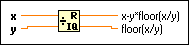# Quotient & Remainder Function

## LabVIEW 2018 Help

Edition Date: March 2018
Part Number: 371361R-01
View Product InfoLabVIEW 2016 HelpLabVIEW 2017 HelpLabVIEW 2018 HelpLabVIEW 2019 HelpLabVIEW 2020 Help

Owning Palette: Numeric Functions

Requires: Base Development System

Computes the integer quotient and the remainder of the inputs. This function rounds floor(x/y) to the nearest integer towards -inf.

The connector pane displays the default data types for this polymorphic function.x can be a scalar number, array or cluster of numbers, array of clusters of numbers, and so on.y can be a scalar number, array or cluster of numbers, array of clusters of numbers, and so on.x-y*floor(x/y) is the remainder. This corresponds to the modulo function of text-based programming languages. When y is 1, the remainder is the fractional part of x.floor(x/y) is the integer quotient. If either input is a floating-point number, the quotient is a floating-point number with an integer value. When y is 1, the quotient is the integer part of x.

## Quotient & Remainder Details

If the integer input value of y is zero, the quotient is zero and remainder is dividend x. For floating-point inputs, if y is zero, the quotient is infinity and the remainder defaults to NaN.Note  Not all real numbers can be represented in the ANSI/IEEE standard floating-point numbers. Because of this, LabVIEW may encounter rounding errors and yield unexpected results if you use floating-point numbers with this function. For exact calculations and comparisons, convert floating-point numbers to integers.